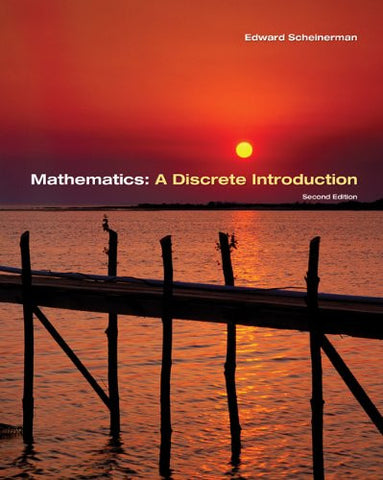# Mathematics: A Discrete Introduction

• \$127.06
• Save \$40
• Binding: Hardcover
• Author: Edward A. Scheinerman
• Publish Date: 2005-06-06

Attention : For textbook, access codes and supplements are not guaranteed with used items.

This book has two primary objectives: It teaches students fundamental concepts in discrete mathematics (from counting to basic cryptography to graph theory), and it teaches students proof-writing skills. With a wealth of learning aids and a clear presentation, the book teaches students not only how to write proofs, but how to think clearly and present cases logically beyond this course. Overall, this book is an introduction to mathematics. In particular, it is an introduction to discrete mathematics. All of the material is directly applicable to computer science and engineering, but it is presented from a mathematician's perspective. While algorithms and analysis appear throughout, the emphasis is on mathematics. Students will learn that discrete mathematics is very useful, especially those whose interests lie in computer science and engineering, as well as those who plan to study probability, statistics, operations research, and other areas of applied mathematics.